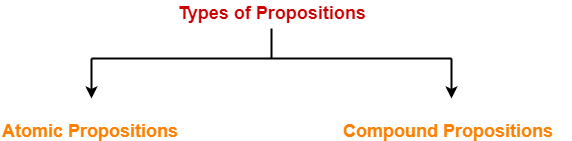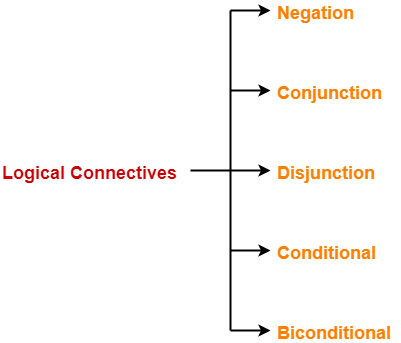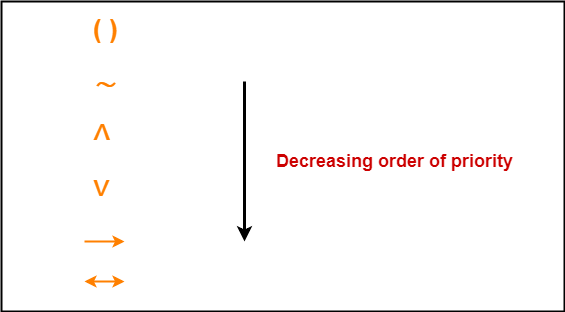## Propositions-

Before you go through this article, make sure that you have gone through the previous article on Propositions.

We have discussed-

• Proposition is a declarative statement that is either true or false but not both.
• Connectives are used to combine the propositions.## Logical Connectives-

 Connectives are the operators that are used to combine one or more propositions.

In propositional logic, there are 5 basic connectives-Name of Connective Connective Word Symbol Negation Not ⌉ or ∼ or ‘ or – Conjunction And ∧ Disjunction Or ∨ Conditional If-then → Biconditional If and only if ↔

## 1. Negation-

If p is a proposition, then negation of p is a proposition which is-

• True when p is false
• False when p is true.

### Truth Table-

 p ∼p F T T F

### Example-

If p : It is raining outside.

Then, Negation of p is-

∼p : It is not raining outside.

## 2. Conjunction-

If p and q are two propositions, then conjunction of p and q is a proposition which is-

• True when both p and q are true
• False when both p and q are false

### Truth Table-

 p q p ∧ q F F F F T F T F F T T T

### Example-

If p and q are two propositions where-

• p : 2 + 4 = 6
• q : It is raining outside.

Then, conjunction of p and q is-

p ∧ q : 2 + 4 = 6 and it is raining outside

## 3. Disjunction-

If p and q are two propositions, then disjunction of p and q is a proposition which is-

• True when either one of p or q or both are true
• False when both p and q are false

### Truth Table-

 p q p ∨ q F F F F T T T F T T T T

### Example-

If p and q are two propositions where-

• p : 2 + 4 = 6
• q : It is raining outside

Then, disjunction of p and q is-

p ∨ q : 2 + 4 = 6 or it is raining outside

## 4. Conditional-

If p and q are two propositions, then-

• Proposition of the type “If p then q” is called a conditional or implication proposition.
• It is true when both p and q are true or when p is false.
• It is false when p is true and q is false.

### Truth Table-

 p q p → q F F T F T T T F F T T T

### Examples-

• If a = b and b = c then a = c.
• If I will go to Australia, then I will earn more money.

## 5. Biconditional-

If p and q are two propositions, then-

• Proposition of the type “p if and only if q” is called a biconditional or bi-implication proposition.
• It is true when either both p and q are true or both p and q are false.
• It is false in all other cases.

### Truth Table-

 p q p ↔ q F F T F T F T F F T T T

### Examples-

• He goes to play a match if and only if it does not rain.
• Birds fly if and only if sky is clear.

## Note-01:

• Negation ≡ NOT Gate of digital electronics.
• Conjunction ≡ AND Gate of digital electronics.
• Disjunction ≡ OR Gate of digital electronics.
• Biconditional = EX-NOR Gate of digital electronics.

## Note-02:

• Each logical connective has some priority.
• This priority order is important while solving questions.
• The decreasing order of priority is-## Note-03:

• Negation, Conjunction, Disjunction and Biconditional are both commutative and associative.
• Conditional is neither commutative nor associative.

To gain better understanding about Logical Connectives,

Watch this Video Lecture

Next Article- Converting English Sentences To Propositional Logic

Get more notes and other study material of Propositional Logic.